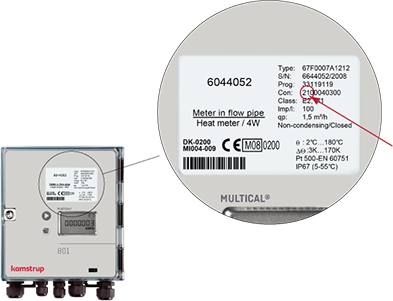#### Interactive User Guides

##### – Heat • Cooling • Water

MULTICAL® 66-CDE

MULTICAL® Compact

MULTICAL® 302

MULTICAL® 401

MULTICAL® 402

MULTICAL® 403

MULTICAL® 601

MULTICAL® 602

MULTICAL® 603

MULTICAL® 801

DDD = 110 / 210 / 410

DDD = 111 / 211 / 411

DDD = 112 / 212 / 412

DDD = 113 / 213 / 413

DDD = 114 / 214 / 414 MVh

DDD = 114 / 214 / 414 GJ

DDD = 115 / 215 / 415

DDD = 116 / 216 / 416

DDD = 117 / 217 / 417

DDD = 118 / 218 / 418

DDD = 119 / 219 / 419

DDD = 120 / 220 / 420

DDD = 121 / 221 / 421

DDD = 122 / 222 / 422

DDD = 123 / 223 / 423

DDD = 124 / 224 / 424

DDD = 125 / 225 / 425

DDD = 126 / 226 / 426

DDD = 127 / 227 / 427

DDD = 128 / 228 / 428

DDD = 129 / 229 / 429

DDD = 130 / 230 / 430

DDD = 131 / 231 / 431

DDD = 132 / 232 / 432

DDD = 133 / 233 / 433

DDD = 134 / 234 / 434

DDD = 137 / 237 / 437

DDD = 142 / 242 / 442

DDD = 143 / 243 / 443

DDD = 156 / 256 / 456

DDD = 157 / 257 / 457

DDD = 158 / 258 / 458

DDD = 182 / 282 / 482

DDD = 183 / 283 / 483

DDD = 238 / 438

DDD = 259

DDD = 510

DDD = 513

DDD = 514

DDD = 515

DDD = 516

DDD = 610

DDD = 611

DDD = 612

DDD = 614

DDD = 615

DDD = 623

DDD = 710 / 810

DDD = 711 / 811

DDD = 712 / 812

DDD = 713 / 813

DDD = 714 / 814

DDD = 756 / 856

DDD = 810

DDD = 811

DDD = 812

DDD = 813

DDD = 814

DDD = 910

DDD = 912

DDD = 913

DDD = 914

DDD = 915

DDD = 916

DDD = 917

DDD = 918

DDD = 919

DDD = 920

DDD = 921

DDD = 922

DDD = 923

DDD = 977

DDD = 978

DDD = 979

DDD = 996

DDD = 997

DDD = 998

MULTICAL® 803

SVM S6

MULTICAL® 61

MULTICAL® 62
– Cold water

MULTICAL® 62
– Hot water

# MULTICAL® 801

## We hope that you will enjoy the product and have a pleasant time.

### How to find your DDD code: